PHY_10_62_V2_P_Faraday law of induction
Оценка 5

# PHY_10_62_V2_P_Faraday law of induction

Оценка 5
ppt
08.05.2020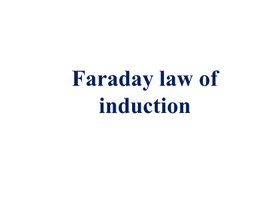PHY_10_62_V2_P_Faraday law of induction.ppt

## Lesson Objectives By the end of the lesson learners will be able: 10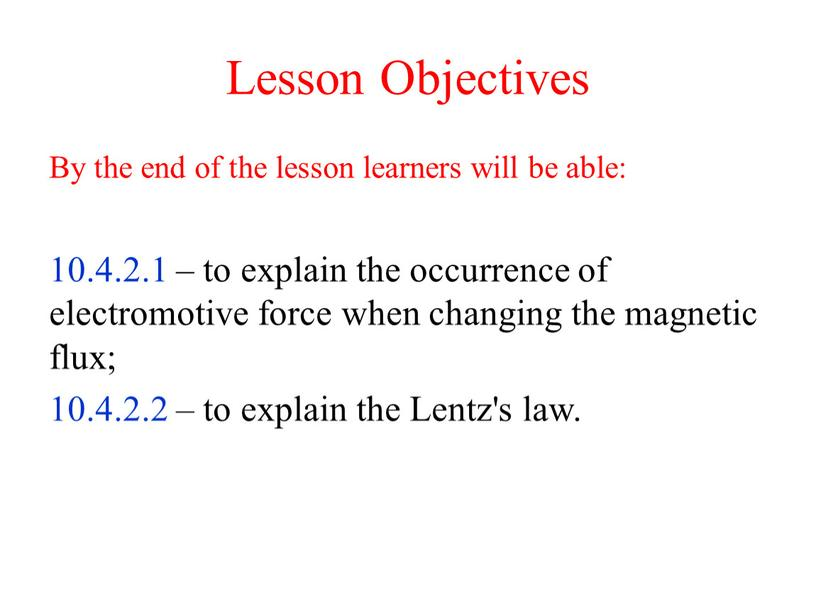Lesson Objectives

By the end of the lesson learners will be able:

10.4.2.1 – to explain the occurrence of electromotive force when changing the magnetic flux;
10.4.2.2 – to explain the Lentz's law.

Induced Voltage and Inductance

Laws of EM Induction

Faraday

Size of the voltage

Lenz

Direction of the voltage

## An EMF IS Induced when: The coil is moved through the magnetic field, across the pole pieces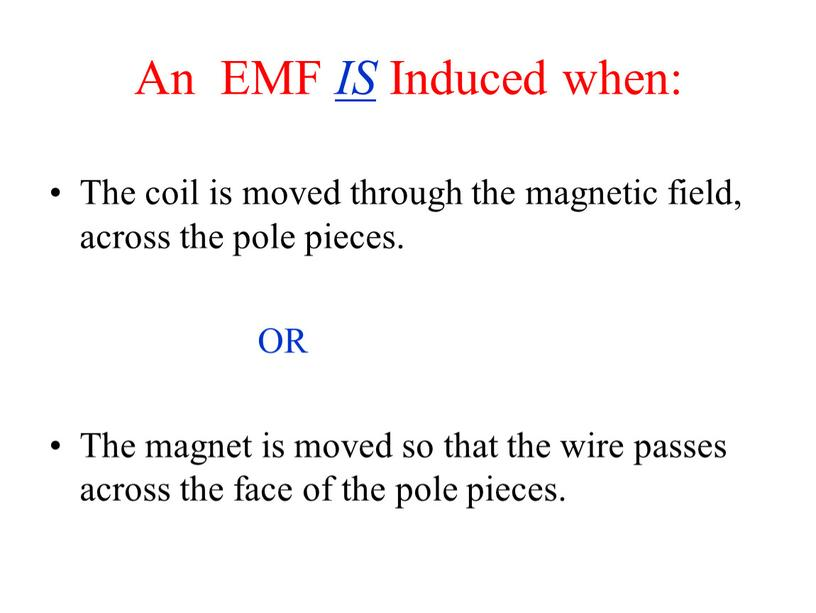An EMF IS Induced when:

The coil is moved through the magnetic field, across the pole pieces.

OR

The magnet is moved so that the wire passes across the face of the pole pieces.

## An EMF is NOT Induced when: The wire is held stationary between the pole-pieces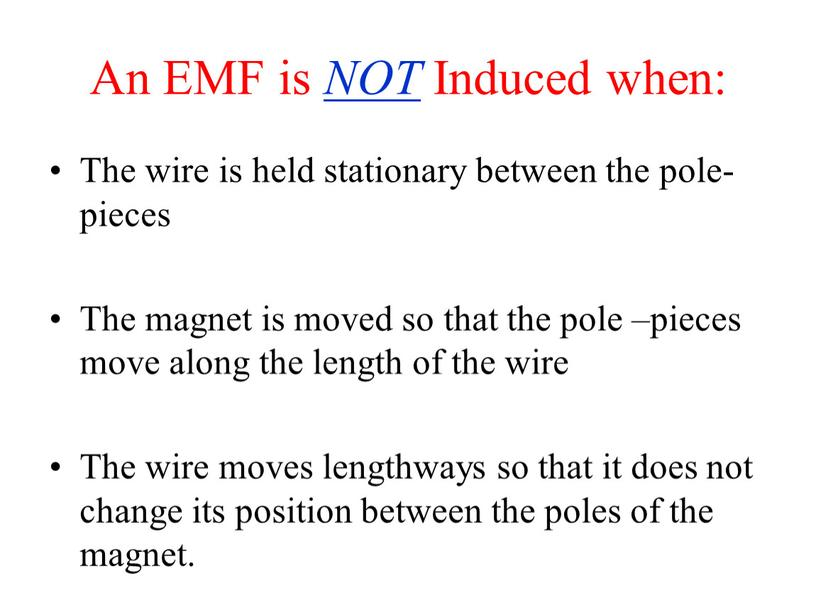An EMF is NOT Induced when:

The wire is held stationary between the pole-pieces

The magnet is moved so that the pole –pieces move along the length of the wire

The wire moves lengthways so that it does not change its position between the poles of the magnet.

## Assessment for Learning 1. Which of the following actions will induce an electromotive force (ℇ) in a conductor? a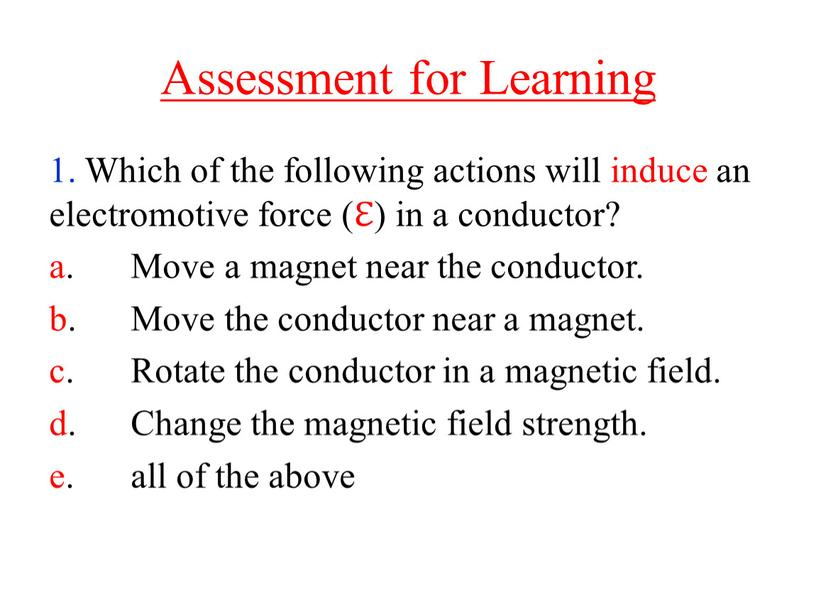Assessment for Learning

1. Which of the following actions will induce an electromotive force (ℇ) in a conductor?
a. Move a magnet near the conductor.
b. Move the conductor near a magnet.
c. Rotate the conductor in a magnetic field.
d. Change the magnetic field strength.
e. all of the above

## Assessment for Learning 2. A circular loop having10 turns with a radius of 29 cm is in a magnetic field that oscillates uniformly between 0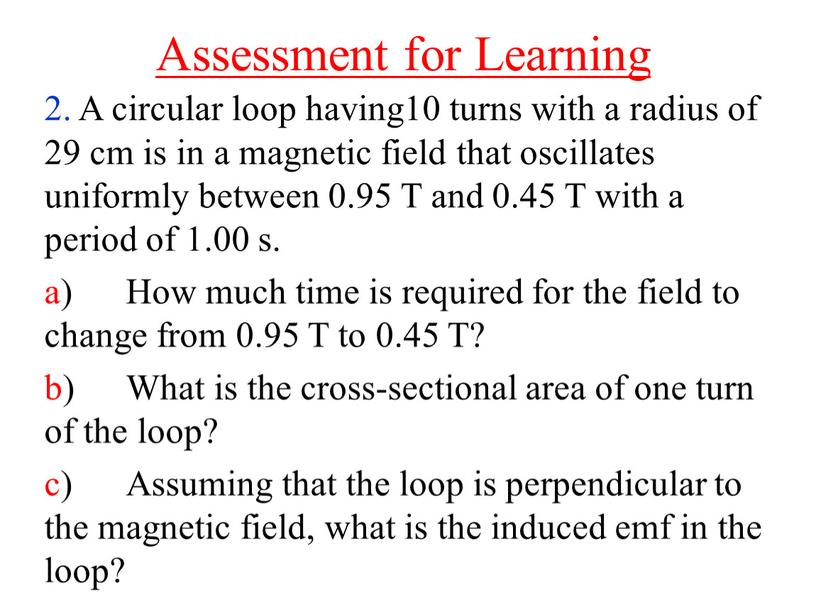Assessment for Learning

2. A circular loop having10 turns with a radius of 29 cm is in a magnetic field that oscillates uniformly between 0.95 T and 0.45 T with a period of 1.00 s.
a) How much time is required for the field to change from 0.95 T to 0.45 T?
b) What is the cross-sectional area of one turn of the loop?
c) Assuming that the loop is perpendicular to the magnetic field, what is the induced emf in the loop?

LENZ’S LAW

THE INDUCED EMF GENERATES A CURRENT THAT SETS UP A MAGNETIC FIELD WHICH ACTS TO OPPOSE THE CHANGE IN MAGNETIC FLUX

B

FLEMING’S RIGHT HAND RULE

conductor

MOTION

PD GENERATED ACROSS A MOVING CONDUCTOR

E = B L v

B INWARDS

20

If the wingspan is L
E = B L v cos θ

Potential Difference across wing tips of an aircraft moving through the Earth’s magnetic field acting at 200 to the vertical.

Скачать файл